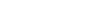# The Role of The Hall Sensor

Jul. 05, 2019

As a Hall Sensor Manufacturer, let's share with you the role of Hall sensors.

1. Line speed measurement

The switch type Hall sensor is regularly placed on the track according to the specified position. When the permanent magnet of the moving vehicle passes the sensor, we can use the measuring circuit to measure the pulse signal, and then the vehicle can be measured according to the distribution of the pulse signal. The speed of movement.

2. Force measurement

If the parameters such as pressure and tension are used as displacement, we can measure the magnitude of the pressure and tension, and the force sensor can be fabricated according to this principle.3. Angular velocity measurement

Generally, a magnetic steel is adhered to the periphery of the disc of non-magnetic material. When the Hall sensor approaches the periphery of the disc, the disc rotates once, and the Hall sensor outputs a pulse at the same time. The rotation can be measured by this principle. The number of turns, if you insert a frequency meter in it, you can measure the speed of rotation.

4. Displacement measurement

It can be used for displacement measurement. The Linear Hall Sensor is placed between two relatively permanent magnets of the same polarity. At this point, this point can be regarded as the displacement zero point, and the magnetic induction intensity is zero. When the sensor is displaced to ΔZ on the Z axis, the sensor will have a voltage output. The magnitude of the displacement is proportional to the voltage.

Technical Support:| Sitemap |